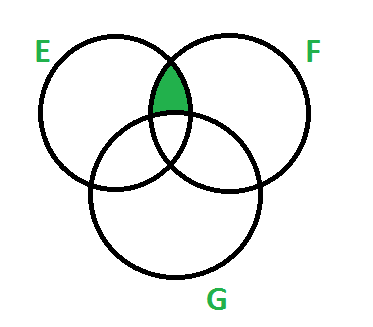# GATE | GATE-CS-2006 | Question 22

Let E, F and G be finite sets.
Let X = (E ∩ F) – (F ∩ G) and Y = (E – (E ∩ G)) – (E – F).
Which one of the following is true?

(A) X ⊂ Y
(B) X ⊃ Y
(C) X = Y
(D) X – Y ≠ φ and Y – X ≠ φ

Explanation: If we draw the venn diagrams of both X and Y, we find that both cover exactly same region (shown in gigure below).So option (C) is correct.

My Personal Notes arrow_drop_up

Article Tags :

Be the First to upvote.

Please write to us at contribute@geeksforgeeks.org to report any issue with the above content.